## Resource of the week: scalene triangles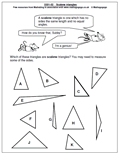This week we take another look at types of triangle. A lot of work is done on triangles in Primary Schools and by year 5 and 6 most children are familiar with the properties of right angled triangles, equilateral triangles and isosceles triangles. An equilateral triangle has all three sides equal and all three angles equal; an isosceles triangle has two equal sides and two equal angles. However, fewer children know about the scalene triangle. A scalene triangle is one which has no sides the same length and no equal angles. Right angled triangles could also be scalene if all the sides are different lengths.

Thanks to MathSphere Ltd for this worksheet: and there are many more similar pages, both on their site and part of the ‘It’s All Figured Out’ CD.

Go to our year 5 shape resources

## Year 2: estimating on a number line 0-10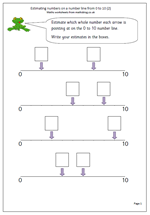By year 2 children will be beginning to understand the vocabulary of estimating, which includes other terms such as roughly, guess how many, about the same as, nearly, close to etc.

This worksheet looks  at estimating a whole number on a number line from 0 to 10. Probably the best technique to use is to try and estimate the middle of the line, which will be 5 and then work either from 0 to 5 or 5 to 10. This is not as easy as it might appear, but after a few goes it does become much less tricky.

One use for this type of activity will come later when children need to interpret graphs where the numbers on the axes go up in fours, fives or tens and intermediate numbers need to be found.

Estimating on a number line (2)

## Resource of the Week: year 4 multiplication and addition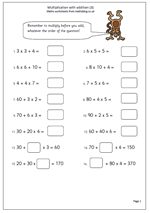This week we are highlighting an important part of year 4 maths which looks at the order of operations.

The rule is that multiplication should be worked out before the addition and in most of these questions that is fairly straightforward. However the questions towards the end are quite tricky.

Let’s look at 70 + ?? x 4 = 150

The best way to go about this is to go in reverse order; subtract 70 from 150 which leaves 80.

Then work out what number multiplied by 4 makes 80, which is 20.

Therefore the missing number is 20.

It is best to check this by going back to the original question; multiply 20 by 4 = 80 and then add 80 and 70 = 150, making the statement true.

Many children will find these very difficult to work out as they require several steps.

## Year 6: solve problems involving money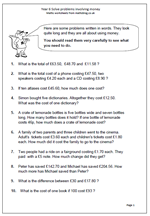By year 6 children should be able to read and understand problems written in prose that include some elements of real life involving money.

They should be able to read the problem, understand the situation described, be able to see what processes are needed to solve it and then lay out working and answers clearly.

Children do find word problems far harder than just being given a ‘sum’ to do and it is important to discuss with them the best way to lay out any working.

One or two of these questions could be done mentally, but most need more than one process to reach the answer. All involve money and are an excellent way of working with two places after the decimal point.

Solve word problems involving money

## Key Stage 2 SAT questions on time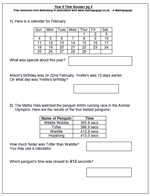Time questions are very popular with the SAT test writers and this page shows some typical examples, using tables and charts.

Children often get confused by questions which say that an event was earlier or later when looking at a calendar. If it was earlier then the number of days have to be subracted. On a calendar moving up one position will subtract 7 days – useful when counting back.

Many more time questions can be found in the year 6 category, under Key Stage 2 Maths SAT Practice.
KS2 SAT maths worksheet: Time (p 4)

## Year 1 maths worksheet: solve simple word problems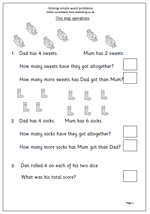One of the targets for Year 1 children is to solve simple word problems. Of course this can be very tricky for children who have yet to develop sufficient reading skills to work out what questions are being asked! Because of this many children will need help with either reading the questions of having the questions read to them.

This page also gives visual clues and again, practical apparatus such as real sweets or socks can be a great help before moving on to just having the written question.

Addition and subtraction are the two operations used here.

Solve simple word problems

## Dividing by 3 table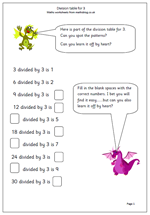In recent years there has been a call for children to not only learn the times tables but also the equivalent division tables. Of course this is the inverse of the 3x table and for children who know this there should not be much of a problem learning this. There are advantages to be had as it can lead to quicker mental arithmetic when carrying out written long and short division. So, here have have a quick look at learning facts connected to dividing by three and an exercise putting this knowledge into practice.

Divide by 3 table